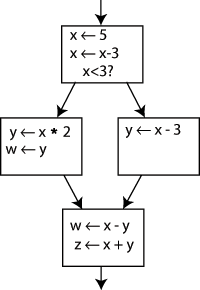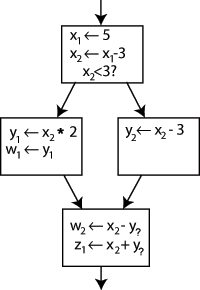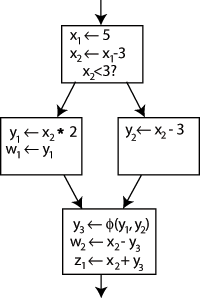# LLVM SSA 介绍

## SSA 介绍

### 1 概念

In compiler design, static single assignment form (often abbreviated as SSA form or simply SSA) is a property of an intermediate representation (IR), which requires that each variable is assigned exactly once, and every variable is defined before it is used.

– From Wikipedia

### 2 为什么要使用 SSA ？

SSA 通过简化程序中变量的特性，可以同时达到两种目的：第一，可以简化很多编译优化方法的过程；第二，对很多编译优化方法来说，可以获得更好的优化结果。下面给出一个例子：

 y := 1
y := 2
x := y

 y1 := 1
y2 := 2
x1 := y2

### 3 如何生成 SSA ？### 4 如何插入 Φ 函数 ？

#### 4.1 求解支配边界

1 for each node b
2   if the number of immediate predecessors of b ≥ 2
3     for each p in immediate predecessors of b
4       runner := p
5       while runner ≠ idom(b)
6         add b to runner’s dominance frontier set
7         runner := idom(runner)

#### 4.2 Φ 函数插入算法

// has-phi(B) is true if a φ-function has already been placed in B
// processed(B) is true if B has already been processed once for variable v
// Assignment-nodes(v) is the set of nodes containing statements assigning to v
// W is the work list (i.e., queue)
function Place-phi-function(v)
for all nodes B in the flow graph do
has-phi(B) = false;
processed(B) = false;
end for
W = ∅;
for all nodes B ∈ Assignment-nodes(v) do
processed(B) = true;
end for
while W != ∅ do
begin
B = Remove(W);
for all nodes y ∈ DF(B) do
if (not has-phi(y)) then
begin
place < v = φ(v, v, ..., v) > in y;
has-phi(y) = true;
if (not processed(y)) then
begin
processed(y) = true;
end
end
end for
end
end

#### 4.3 重命名算法

begin // calling program
for all variables x in the flow graph do
V = ∅;
v = 1;
push 0 onto V;
Rename-variables(x, Start);
end for
end

function Rename-variables(x, B)  // x is a variable and B is a block
begin
ve = Top(V);  // V is the version stack of x
for all statements s ∈ B do
if s is a non-φ statement then
replace all uses of x in the RHS(s) with Top(V);
if s defines x then
begin
replace x with xv in its definition;
push xv onto V;  // xv is the renamed version of x in this definition
v = v + 1;  // v is the version number counter
end
end for

for all successors s of B in the flow graph do
j = predecessor index of B with respect to s
for all φ-functions f in s which define x do
replace the jth operand of f with Top(V);
end for
end for
for all children c of B in the dominator tree do  // Depth-First Order
Rename-variables(x, c);
end for
repeat Pop(V);
until (Top(V) == ve);
end

## LLVM 里的 SSA

### 1 LLVM IR

int foo(int a, int b, int e) {
int c, d;
goto L1;

L1: {
c = a + b;
d = c - a;
if (d) goto L2;
d = b * d;
e = e + 1;
}
L2: {
b = a + b;
e = c - a;
if (e) goto L1;
a = b * d;
b = a - d;
}
return b;
}

; ModuleID = '.\test.c'
target datalayout = "e-m:x-p:32:32-i64:64-f80:32-n8:16:32-a:0:32-S32"
target triple = "i686-pc-windows-msvc18.0.0"

; Function Attrs: nounwind
define i32 @foo(i32 %a, i32 %b, i32 %e) #0 {
entry:
%e.addr = alloca i32, align 4
%b.addr = alloca i32, align 4
%a.addr = alloca i32, align 4
%c = alloca i32, align 4
%d = alloca i32, align 4
store i32 %e, i32* %e.addr, align 4
store i32 %b, i32* %b.addr, align 4
store i32 %a, i32* %a.addr, align 4
br label %L1

L1:                                               ; preds = %if.then5, %entry
store i32 %add, i32* %c, align 4
%2 = load i32, i32* %c, align 4
%sub = sub nsw i32 %2, %3
store i32 %sub, i32* %d, align 4
%4 = load i32, i32* %d, align 4
%tobool = icmp ne i32 %4, 0
br i1 %tobool, label %if.then, label %if.end

if.then:                                          ; preds = %L1
br label %L2

if.end:                                           ; preds = %L1
%6 = load i32, i32* %d, align 4
%mul = mul nsw i32 %5, %6
store i32 %mul, i32* %d, align 4
br label %L2

L2:                                               ; preds = %if.end, %if.then
%10 = load i32, i32* %c, align 4
%sub3 = sub nsw i32 %10, %11
store i32 %sub3, i32* %e.addr, align 4
%tobool4 = icmp ne i32 %12, 0
br i1 %tobool4, label %if.then5, label %if.end6

if.then5:                                         ; preds = %L2
br label %L1

if.end6:                                          ; preds = %L2
%14 = load i32, i32* %d, align 4
%mul7 = mul nsw i32 %13, %14
store i32 %mul7, i32* %a.addr, align 4
%16 = load i32, i32* %d, align 4
%sub8 = sub nsw i32 %15, %16
store i32 %sub8, i32* %b.addr, align 4
ret i32 %17
}

attributes #0 = { nounwind "disable-tail-calls"="false" "less-precise-fpmad"="false" "no-frame-pointer-elim"="true" "no-frame-pointer-elim-non-leaf" "no-infs-fp-math"="false" "no-nans-fp-math"="false" "stack-protector-buffer-size"="8" "target-cpu"="pentium4" "target-features"="+fxsr,+mmx,+sse,+sse2" "unsafe-fp-math"="false" "use-soft-float"="false" }

!llvm.ident = !{!0}

!0 = !{!"clang version 3.8.0 (tags/RELEASE_380/final)"}


LLVM does require all register values to be in SSA form, it does not require (or permit) memory objects to be in SSA form.

### 2 LLVM 的内存

...
entry:
%c = alloca i32, align 4
...
L1:
store i32 %add, i32* %c, align 4
...
L2:
%10 = load i32, i32* %c, align 4
...

1. Each mutable variable becomes a stack allocation.
2. Each read of the variable becomes a load from the stack.
3. Each update of the variable becomes a store to the stack.
4. Taking the address of a variable just uses the stack address directly.

### 3 问题总结

c = a + b;
c = c - b;

%a = alloca i32, align4
%b = alloca i32, align4
%c = alloca i32, align4
%0 = load i32, i32* %a, align 4
%1 = load i32, i32* %b, align 4
store i32 %add, i32* %c, align 4
%2 = load i32, i32* %c, align 4
%3 = load i32, i32* %b, align 4
%sub = sub nsw i32 %2, %3
store i32 %sub, i32* %c, align 4

; ModuleID = '.\test.c'
target datalayout = "e-m:x-p:32:32-i64:64-f80:32-n8:16:32-a:0:32-S32"
target triple = "i686-pc-windows-msvc18.0.0"

; Function Attrs: norecurse nounwind readnone
define i32 @foo(i32 %a, i32 %b, i32 %e) #0 {
entry:
br label %L1

L1:                                               ; preds = %L1, %entry
%b.addr.0 = phi i32 [ %b, %entry ], [ %add, %L1 ]
%tobool = icmp eq i32 %b.addr.0, 0
br i1 %tobool, label %if.end6, label %L1

if.end6:                                          ; preds = %L1
%sub8 = sub nsw i32 %mul7, %b.addr.0
ret i32 %sub8
}

attributes #0 = { norecurse nounwind readnone "disable-tail-calls"="false" "less-precise-fpmad"="false" "no-frame-pointer-elim"="false" "no-infs-fp-math"="false" "no-nans-fp-math"="false" "stack-protector-buffer-size"="8" "target-cpu"="pentium4" "target-features"="+fxsr,+mmx,+sse,+sse2" "unsafe-fp-math"="false" "use-soft-float"="false" }

!llvm.ident = !{!0}

!0 = !{!"clang version 3.8.0 (tags/RELEASE_380/final)"}

## 参考文献

12-07421
11-188457
01-22197
09-191万+
01-161384
11-24310
11-215763
09-19993
12-03916
06-115292
06-24436
12-2594
04-02500
03-08389
12-161951
08-29548
03-282005
04-263853
06-101631
12-292926

### “相关推荐”对你有帮助么？

•非常没帮助
•没帮助
•一般
•有帮助
•非常有帮助Enorsee

¥2 ¥4 ¥6 ¥10 ¥20余额支付 (余额：-- )扫码支付获取中扫码支付点击重新获取扫码支付1.余额是钱包充值的虚拟货币，按照1:1的比例进行支付金额的抵扣。
2.余额无法直接购买下载，可以购买VIP、C币套餐、付费专栏及课程。余额充值